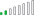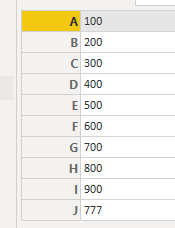cancel
Showing results for
Did you mean:Resolver II

## Create an array from table (Power Query)

Hi guys!

I'm attempting to create an array that has one single input to one single output coming from a table. I currently have a table with multiple columns and I want to map two columns together.

A sample table is supplied below:

``````let
Source = Table.FromRows(Json.Document(Binary.Decompress(Binary.FromText("LYu3DcAwDMB+0ezBXfaYXl4w/P8bYQANBMiBY0gQJwsE72W6IRFfIVonfINknfEdsnXBDyjWFT+hWit+gVo3/IZm3fEHunXwxPsPqjLnBw==", BinaryEncoding.Base64), Compression.Deflate)), let _t = ((type nullable text) meta [Serialized.Text = true]) in type table [Column1 = _t, Column2 = _t, Column3 = _t])
in
Source``````

The sample input looks like this:Considering I may have 1000+ records, I want to be able to create an array with the expected output where I select and map Column2 to Column3.

EDIT: Considering all items from Column2 and Column3 will be unique

``````let
Source = Table.FromRows(Json.Document(Binary.Decompress(Binary.FromText("LYu3DcAwDMB+0ezBXfaYXl4w/P8bYQANBMiBY0gQJwsE72W6IRFfIVonfINknfEdsnXBDyjWFT+hWit+gVo3/IZm3fEHunXwxPsPqjLnBw==", BinaryEncoding.Base64), Compression.Deflate)), let _t = ((type nullable text) meta [Serialized.Text = true]) in type table [Column1 = _t, Column2 = _t, Column3 = _t]),
ExpectedResult =
[
A = 100,
B = 200,
C = 300,
D = 400,
E = 500,
F = 600,
G = 700,
H = 800,
I = 900,
J = 777
]
in
ExpectedResult``````How would this be possible? I think I've done it before but I'm currently having a brain fart.

1 ACCEPTED SOLUTIONMicrosoft

Here is a modified version of your query that does this.

``````let
Source = Table.FromRows(Json.Document(Binary.Decompress(Binary.FromText("LYu3DcAwDMB+0ezBXfaYXl4w/P8bYQANBMiBY0gQJwsE72W6IRFfIVonfINknfEdsnXBDyjWFT+hWit+gVo3/IZm3fEHunXwxPsPqjLnBw==", BinaryEncoding.Base64), Compression.Deflate)), let _t = ((type nullable text) meta [Serialized.Text = true]) in type table [Column1 = _t, Column2 = _t, Column3 = _t]),
ToRecords = Table.ToRecords(Source),
Custom1 = List.Accumulate(ToRecords, [], (current, next) => Record.AddField(current, next[Column2], next[Column3]))
in
Custom1``````
2 REPLIES 2Microsoft

Here is a modified version of your query that does this.

``````let
Source = Table.FromRows(Json.Document(Binary.Decompress(Binary.FromText("LYu3DcAwDMB+0ezBXfaYXl4w/P8bYQANBMiBY0gQJwsE72W6IRFfIVonfINknfEdsnXBDyjWFT+hWit+gVo3/IZm3fEHunXwxPsPqjLnBw==", BinaryEncoding.Base64), Compression.Deflate)), let _t = ((type nullable text) meta [Serialized.Text = true]) in type table [Column1 = _t, Column2 = _t, Column3 = _t]),
ToRecords = Table.ToRecords(Source),
Custom1 = List.Accumulate(ToRecords, [], (current, next) => Record.AddField(current, next[Column2], next[Column3]))
in
Custom1``````Resolver II

@artemus Thank you! I attempted to use List.Accumulate but failed lol. This is working perfectly! Cheers!